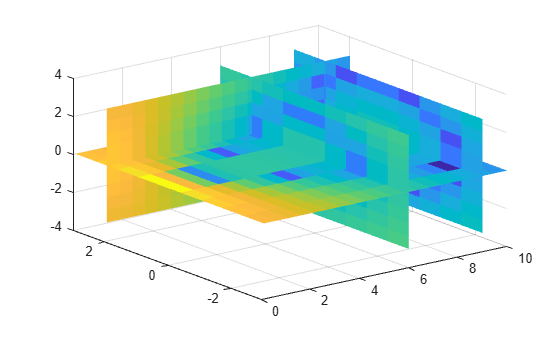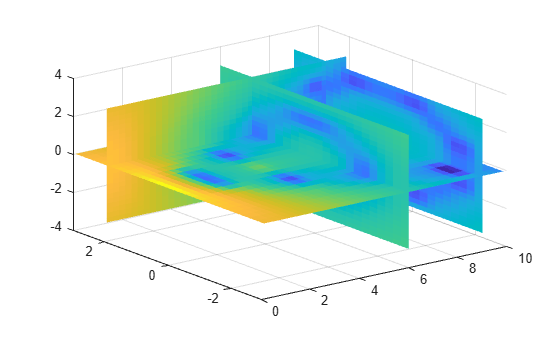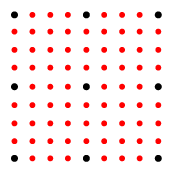# interp3

Interpolation for 3-D gridded data in meshgrid format

## Syntax

``Vq = interp3(X,Y,Z,V,Xq,Yq,Zq)``
``Vq = interp3(V,Xq,Yq,Zq)``
``Vq = interp3(V)``
``Vq = interp3(V,k)``
``Vq = interp3(___,method)``
``Vq = interp3(___,method,extrapval)``

## Description

example

````Vq = interp3(X,Y,Z,V,Xq,Yq,Zq)` returns interpolated values of a function of three variables at specific query points using linear interpolation. The results always pass through the original sampling of the function. `X`, `Y`, and `Z` contain the coordinates of the sample points. `V` contains the corresponding function values at each sample point. `Xq`, `Yq`, and `Zq` contain the coordinates of the query points. ```
````Vq = interp3(V,Xq,Yq,Zq)` assumes a default grid of sample points. The default grid points cover the region, `X=1:n`, `Y=1:m`, `Z=1:p`, where `[m,n,p] = size(V)`. Use this syntax when you want to conserve memory and are not concerned about the absolute distances between points.```
````Vq = interp3(V)` returns the interpolated values on a refined grid formed by dividing the interval between sample values once in each dimension.```
````Vq = interp3(V,k)` returns the interpolated values on a refined grid formed by repeatedly halving the intervals `k` times in each dimension. This results in `2^k-1` interpolated points between sample values.```

example

````Vq = interp3(___,method)` specifies an alternative interpolation method: `'linear'`, `'nearest'`, `'cubic'`, `'makima'`, or `'spline'`. The default method is `'linear'`.```

example

````Vq = interp3(___,method,extrapval)` also specifies `extrapval`, a scalar value that is assigned to all queries that lie outside the domain of the sample points. If you omit the `extrapval` argument for queries outside the domain of the sample points, then based on the `method` argument `interp3` returns one of the following: The extrapolated values for the `'spline'` and `'makima'` methods`NaN` values for other interpolation methods ```

## Examples

collapse all

Load the points and values of the flow function, sampled at 10 points in each dimension.

`[X,Y,Z,V] = flow(10);`

The `flow` function returns the grid in the arrays, `X`, `Y`, `Z`. The grid covers the region, $0.1\le X\le 10$, $-3\le Y\le 3$, $-3\le Z\le 3$, and the spacing is $\Delta X=0.5$, $\Delta Y=0.7$, and $\Delta Z=0.7$.

Now, plot slices through the volume of the sample at: `X=6`, `X=9`, `Y=2`, and `Z=0`.

```figure slice(X,Y,Z,V,[6 9],2,0); shading flat```Create a query grid with spacing of 0.25.

`[Xq,Yq,Zq] = meshgrid(.1:.25:10,-3:.25:3,-3:.25:3);`

Interpolate at the points in the query grid and plot the results using the same slice planes.

```Vq = interp3(X,Y,Z,V,Xq,Yq,Zq); figure slice(Xq,Yq,Zq,Vq,[6 9],2,0); shading flat```Load the points and values of the flow function, sampled at 10 points in each dimension.

`[X,Y,Z,V] = flow(10);`

The `flow` function returns the grid in the arrays, `X`, `Y`, `Z`. The grid covers the region, $0.1\le X\le 10$, $-3\le Y\le 3$, $-3\le Z\le 3$, and the spacing is $\Delta X=0.5$, $\Delta Y=0.7$, and $\Delta Z=0.7$.

Plot slices through the volume of the sample at: `X=6`, `X=9`, `Y=2`, and `Z =0`.

```figure slice(X,Y,Z,V,[6 9],2,0); shading flat```Create a query grid with spacing of 0.25.

`[Xq,Yq,Zq] = meshgrid(.1:.25:10,-3:.25:3,-3:.25:3);`

Interpolate at the points in the query grid using the `'cubic'` interpolation method. Then plot the results.

```Vq = interp3(X,Y,Z,V,Xq,Yq,Zq,'cubic'); figure slice(Xq,Yq,Zq,Vq,[6 9],2,0); shading flat```Create the grid vectors, `x`, `y`, and `z`. These vectors define the points associated with values in `V`.

```x = 1:100; y = (1:50)'; z = 1:30;```

Define the sample values to be a 50-by-100-by-30 random number array, `V`. Use the `rand` function to create the array.

```rng('default') V = rand(50,100,30);```

Evaluate `V` at three points outside the domain of `x`, `y`, and `z`. Specify `extrapval = -1`.

```xq = [0 0 0]; yq = [0 0 51]; zq = [0 101 102]; vq = interp3(x,y,z,V,xq,yq,zq,'linear',-1)```
```vq = 1×3 -1 -1 -1 ```

All three points evaluate to `-1` because they are outside the domain of `x`, `y`, and `z`.

## Input Arguments

collapse all

Sample grid points, specified as real arrays or vectors. The sample grid points must be unique.

• If `X`, `Y`, and `Z` are arrays, then they contain the coordinates of a full grid (in meshgrid format). Use the `meshgrid` function to create the `X`, `Y`, and `Z` arrays together. These arrays must be the same size.

• If `X`, `Y`, and `Z` are vectors, then they are treated as a grid vectors. The values in these vectors must be strictly monotonic, either increasing or decreasing.

Example: `[X,Y,Z] = meshgrid(1:30,-10:10,1:5)`

Data Types: `single` | `double`

Sample values, specified as a real or complex array. The size requirements for `V` depend on the size of `X`, `Y`, and `Z`:

• If `X`, `Y`, and `Z` are arrays representing a full grid (in `meshgrid` format), then the size of `V` matches the size of `X`, `Y`, or `Z` .

• If `X`, `Y`, and `Z` are grid vectors, then `size(V) = [length(Y) length(X) length(Z)]`.

If `V` contains complex numbers, then `interp3` interpolates the real and imaginary parts separately.

Example: `rand(10,10,10)`

Data Types: `single` | `double`
Complex Number Support: Yes

Query points, specified as a real scalars, vectors, or arrays.

• If `Xq`, `Yq`, and `Zq` are scalars, then they are the coordinates of a single query point in R3.

• If `Xq`, `Yq`, and `Zq` are vectors of different orientations, then `Xq`, `Yq`, and `Zq` are treated as grid vectors in R3.

• If `Xq`, `Yq`, and `Zq` are vectors of the same size and orientation, then `Xq`, `Yq`, and `Zq` are treated as scattered points in R3.

• If `Xq`, `Yq`, and `Zq` are arrays of the same size, then they represent either a full grid of query points (in `meshgrid` format) or scattered points in R3.

Example: `[Xq,Yq,Zq] = meshgrid((1:0.1:10),(-5:0.1:0),3:5)`

Data Types: `single` | `double`

Refinement factor, specified as a real, nonnegative, integer scalar. This value specifies the number of times to repeatedly divide the intervals of the refined grid in each dimension. This results in `2^k-1` interpolated points between sample values.

If `k` is `0`, then `Vq` is the same as `V`.

`interp3(V,1)` is the same as `interp3(V)`.

The following illustration depicts `k=2` in one plane of R3. There are 72 interpolated values in red and 9 sample values in black.Example: `interp3(V,2)`

Data Types: `single` | `double`

Interpolation method, specified as one of the options in this table.

`'linear'`The interpolated value at a query point is based on linear interpolation of the values at neighboring grid points in each respective dimension. This is the default interpolation method.C0
• Requires at least two grid points in each dimension

• Requires more memory than `'nearest'`

`'nearest'`The interpolated value at a query point is the value at the nearest sample grid point. Discontinuous
• Requires two grid points in each dimension

• Fastest computation with modest memory requirements

`'cubic'`The interpolated value at a query point is based on a cubic interpolation of the values at neighboring grid points in each respective dimension. The interpolation is based on a cubic convolution.C1
• Grid must have uniform spacing in each dimension, but the spacing does not have to be the same for all dimensions

• Requires at least four points in each dimension

• Requires more memory and computation time than `'linear'`

`'makima'`Modified Akima cubic Hermite interpolation. The interpolated value at a query point is based on a piecewise function of polynomials with degree at most three evaluated using the values of neighboring grid points in each respective dimension. The Akima formula is modified to avoid overshoots.C1
• Requires at least 2 points in each dimension

• Produces fewer undulations than `'spline'`

• Computation time is typically less than `'spline'`, but the memory requirements are similar

`'spline'`The interpolated value at a query point is based on a cubic interpolation of the values at neighboring grid points in each respective dimension. The interpolation is based on a cubic spline using not-a-knot end conditions.C2
• Requires four points in each dimension

• Requires more memory and computation time than `'cubic'`

Function value outside domain of `X`, `Y`, and `Z`, specified as a real or complex scalar. `interp3` returns this constant value for all points outside the domain of `X`, `Y`, and `Z`.

Example: `5`

Example: `5+1i`

Data Types: `single` | `double`
Complex Number Support: Yes

## Output Arguments

collapse all

Interpolated values, returned as a real or complex scalar, vector, or array. The size and shape of `Vq` depends on the syntax you use and, in some cases, the size and value of the input arguments.

SyntaxesSpecial ConditionsSize of VqExample
`interp3(X,Y,Z,V,Xq,Yq,Zq)`
`interp3(V,Xq,Yq,Zq)`
and variations of these syntaxes that include `method` or `extrapval`
`Xq`, `Yq`, and `Zq` are scalars.Scalar`size(Vq) = [1 1]` when you pass `Xq`, `Yq`, and `Zq` as scalars.
Same as above`Xq`, `Yq`, and `Zq` are vectors of the same size and orientation.Vector of same size and orientation as `Xq`, `Yq`, and `Zq`If `size(Xq) = [100 1]`,
and ```size(Yq) = [100 1]```,
and ```size(Zq) = [100 1]```,
then `size(Vq) = [100 1]`.
Same as above`Xq`, `Yq`, and `Zq` are vectors of mixed orientation.`size(Vq) = [length(Y) length(X) length(Z)]`If `size(Xq) = [1 100]`,
and ```size(Yq) = [50 1]```,
and `size(Zq) = [1 5]`,
then `size(Vq) = [50 100 5]`.
Same as above`Xq`, `Yq`, and `Zq` are arrays of the same size.Array of the same size as `Xq`, `Yq`, and `Zq`If `size(Xq) = [50 25]`,
and ```size(Yq) = [50 25]```,
and `size(Zq) = [50 25]`,
then `size(Vq) = [50 25]`.
`interp3(V,k)`
and variations of this syntax that include `method` or `extrapval`
None

Array in which the length of the `i`th dimension is
`2^k * (size(V,i)-1)+1`

If `size(V) = [10 12 5]`,
and ```k = 3```,
then `size(Vq) = [73 89 33]`.

collapse all

### Strictly Monotonic

A set of values that are always increasing or decreasing, without reversals. For example, the sequence, ```a = [2 4 6 8]``` is strictly monotonic and increasing. The sequence, ```b = [2 4 4 6 8]``` is not strictly monotonic because there is no change in value between `b(2)` and `b(3)`. The sequence, `c = [2 4 6 8 6]` contains a reversal between `c(4)` and `c(5)`, so it is not monotonic at all.

### Full Grid (in meshgrid Format)

For `interp3`, a full grid consists of three arrays whose elements represent a grid of points that define a region in R3. The first array contains the x-coordinates, the second array contains the y-coordinates, and the third array contains the z-coordinates. The values in each array vary along a single dimension and are constant along the other dimensions.

The values in the x-array are strictly monotonic, increasing, and vary along the second dimension. The values in the y-array are strictly monotonic, increasing, and vary along the first dimension. The values in the z-array are strictly monotonic, increasing, and vary along the third dimension. Use the `meshgrid` function to create a full grid that you can pass to `interp3`.

### Grid Vectors

For `interp3`, grid vectors consist of three vectors of mixed-orientation that define the points on a grid in R3.

For example, the following code creates the grid vectors for the region, 1 ≤ x ≤ 3, 4 ≤ y ≤ 5, and 6 ≤ z ≤ 8:

```x = 1:3; y = (4:5)'; z = 6:8;```

### Scattered Points

For `interp3`, scattered points consist of three arrays or vectors, `Xq`, `Yq`, and `Zq`, that define a collection of points scattered in R3. The ith array contains the coordinates in the ith dimension.

For example, the following code specifies the points, (1, 19, 10), (6, 40, 1), (15, 33, 22), and (0, 61, 13).

```Xq = [1 6; 15 0]; Yq = [19 40; 33 61]; Zq = [10 1; 22 13];```

## Version History

Introduced before R2006a